# Identify Key Parameters for Estimation (Code)

This example shows how to use sensitivity analysis to narrow down the number of parameters that you need to estimate to fit a model. This example uses a model of the vestibulo-ocular reflex, which generates compensatory eye movements.

### Model Description

The vestibulo-ocular reflex (VOR) enables the eyes to move at the same speed and in the opposite direction as the head, so that vision is not blurred when the head moves during normal activity. For example, if the head turns in one direction, the eyes turn in the opposite direction, with the same speed. This happens even in the dark. In fact, the VOR is most easily characterized by measurements in the dark, to ensure that eye movements are predominantly driven by the VOR.

The file `sdoVOR_Data.mat` contains uniformly sampled data of stimulation and eye movements. If the VOR were perfectly compensatory, then a plot of eye movement data, when flipped vertically, would overlay exactly on top of a plot of head motion data. Such a system would be described by a gain of 1 and a phase of 180 degrees. However, when we plot the data in the file `sdoVOR_Data.mat`, the eye movements are close, but not perfectly compensatory.

```load sdoVOR_Data.mat; % Column vectors: Time HeadData EyeData figure plot(Time, HeadData, ':b', Time, EyeData, '-g') xlabel('Time (sec)') ylabel('Angular Velocity (deg/sec)') ylim([-110 110]) legend('Head Data', 'Eye Data') ```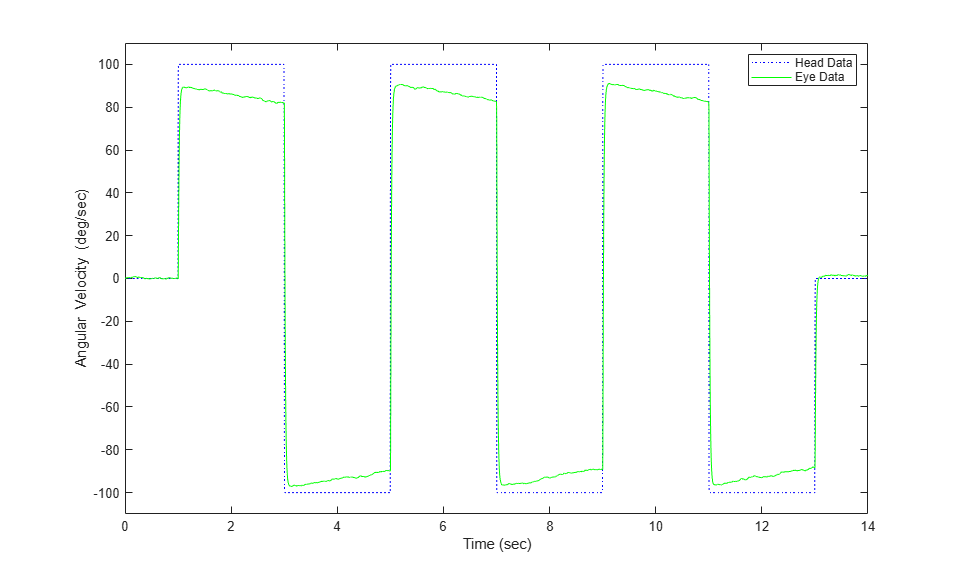The eye movement data does not perfectly overlay the head motion data, and this can be modeled by several factors. Head rotation is sensed by organs in the inner ears, known as semicircular canals. These detect head motion and transmit signals about head motion to the brain, which sends motor commands to the eye muscles, so that eye movements compensate for head motion. We would like to use this eye movement data to estimate the parameters in the models for these various stages. The model we will use is shown below. There are four parameters in the model: `Delay`, `Gain`, `Tc`, and `Tp`.

```model_name = 'sdoVOR'; open_system(model_name) ```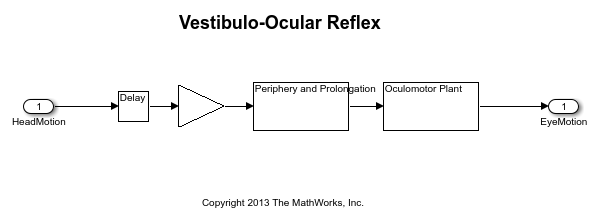The Delay parameter models the fact that there is some delay in communicating the signals from the inner ear to the brain and the eyes. This delay is due to the time needed for chemical neurotransmitters to traverse the synaptic clefts between nerve cells. Based on the number of synapses involved in the vestibulo-ocular reflex, this delay is expected to be around 5 ms. For estimation purposes, we will assume it is between 2 and 9 ms.

```Delay = sdo.getParameterFromModel(model_name, 'Delay'); Delay.Value = 0.005; % seconds Delay.Minimum = 0.002; Delay.Maximum = 0.009; ```

The Gain parameter models the fact that the eyes do not move quite as much as the head does. We will use 0.8 as our initial guess, and assume it is between 0.6 and 1.

```Gain = sdo.getParameterFromModel(model_name, 'Gain'); Gain.Value = 0.8; Gain.Minimum = 0.6; Gain.Maximum = 1; ```

The Tc parameter models the dynamics associated with the semicircular canals, as well as some additional neural processing. The canals are high-pass filters, because after a subject has been put into rotational motion, the neurally active membranes in the canals slowly relax back to resting position, so the canals stop sensing motion. Thus in the plot above, after the stimulation undergoes transition edges, the eye movements tend to depart from the stimulation over time. Based on mechanical characteristics of the canals, combined with additional neural processing which prolongs this time constant to improve the accuracy of the VOR, we will estimate the Tc parameter to be 15 seconds, and assume it is between 10 and 30 seconds.

```Tc = sdo.getParameterFromModel(model_name, 'Tc'); Tc.Value = 15; Tc.Minimum = 10; Tc.Maximum = 30; ```

Finally, the Tp parameter models the dynamics of the oculomotor plant, i.e. the eye and the muscles and tissues attached to it. The plant can be modeled by two poles, however it is believed that the pole with the larger time constant is cancelled by precompensation in the brain, to enable the eye to make quick movements. Thus in the plot, when the stimulation undergoes transition edges, the eye movements follow with only a little delay. For the Tp parameter, we will use 0.01 seconds as our initial guess, and assume it is between 0.005 and 0.05 seconds.

```Tp = sdo.getParameterFromModel(model_name, 'Tp'); Tp.Value = 0.01; Tp.Minimum = 0.005; Tp.Maximum = 0.05; ```

Collect these parameters into a vector.

```v = [Delay Gain Tc Tp]; ```

### Compare Measured Data to Initial Simulated Output

Create an Experiment object. Specify `HeadData` as input.

```Exp = sdo.Experiment(model_name); Exp.InputData = timeseries(HeadData, Time); ```

Associate eye movement data with model output.

```EyeMotion = Simulink.SimulationData.Signal; EyeMotion.Name = 'EyeMotion'; EyeMotion.BlockPath = [model_name '/Oculomotor Plant']; EyeMotion.PortType = 'outport'; EyeMotion.PortIndex = 1; EyeMotion.Values = timeseries(EyeData, Time); ```

Add `EyeMotion` to the experiment.

```Exp.OutputData = EyeMotion; ```

Use the data's timing characteristics in the model.

```stop_time = Time(end); set_param(gcs, 'StopTime', num2str(stop_time)); dt = Time(2) - Time(1); set_param(gcs, 'FixedStep', num2str(dt)) ```

Create a simulation scenario using the experiment, and obtain the simulated output.

```Exp = setEstimatedValues(Exp, v); % use vector of parameters/states Simulator = createSimulator(Exp); Simulator = sim(Simulator); ```

Search for the model_residual signal in the logged simulation data.

```SimLog = find(Simulator.LoggedData, ... get_param(model_name, 'SignalLoggingName') ); EyeSignal = find(SimLog, 'EyeMotion'); ```

The model output does not match the data very well, as shown by the residual, which we can compute by calling the objective function.

```estFcn = @(v) sdoVOR_Objective(v, Simulator, Exp, 'Residuals'); Model_Error = estFcn(v); plot(Time, EyeData, '-g', ... EyeSignal.Values.Time, EyeSignal.Values.Data, '--c', ... Time, Model_Error.F, '-r'); xlabel('Time (sec)'); ylabel('Angular Velocity (deg/sec)'); legend('Eye Data', 'Model', 'Residual'); ```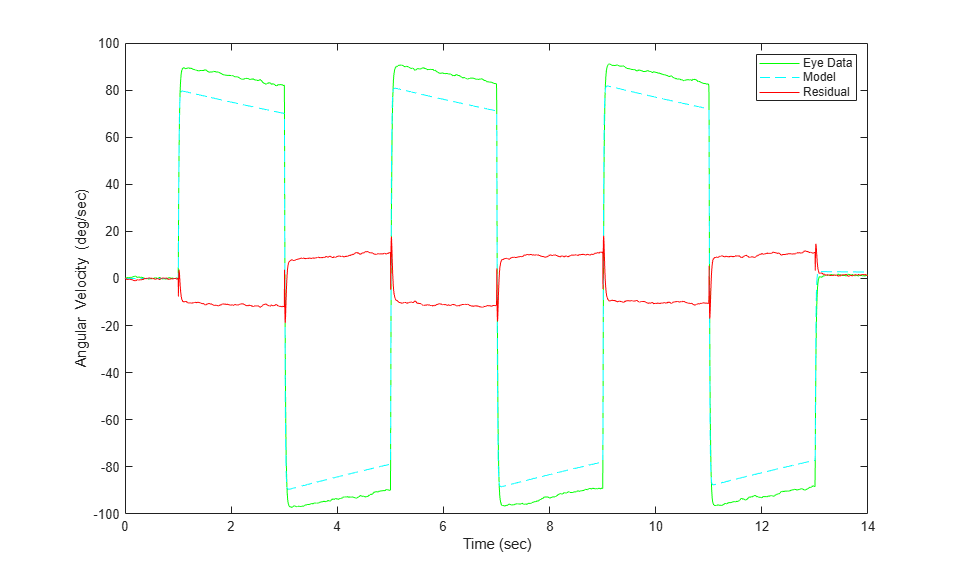The objective function used above is defined in the file "sdoVOR_Objective.m".

```type sdoVOR_Objective.m ```
```function vals = sdoVOR_Objective(v, Simulator, Exp, Method) % Compare model output with data % % Inputs: % v - vector of parameters and/or states % Simulator - used to simulate the model % Exp - Experiment object % Method - 'SSE' for scalar output, 'Residuals' for vector of residuals % Copyright 2014-2015 The MathWorks, Inc. % Requirement setup req = sdo.requirements.SignalTracking; req.Type = '=='; req.Method = Method; % If Residuals requested, keep on same scale as signals, for plotting switch Method case 'Residuals' req.Normalize = 'off'; end % Simulate the model Exp = setEstimatedValues(Exp, v); % use vector of parameters/states Simulator = createSimulator(Exp,Simulator); Simulator = sim(Simulator); % Compare model output with data SimLog = find(Simulator.LoggedData, ... get_param(Exp.ModelName, 'SignalLoggingName') ); OutputModel = find(SimLog, 'EyeMotion'); Model_Error = evalRequirement(req, OutputModel.Values, Exp.OutputData.Values); vals.F = Model_Error; ```

### Sensitivity Analysis

Create an object to sample the parameter space.

```ps = sdo.ParameterSpace([Delay ; Gain ; Tc ; Tp]); ```

Generate 100 samples from the parameter space.

```rng default; % for reproducibility x = sdo.sample(ps, 100); sdo.scatterPlot(x); ```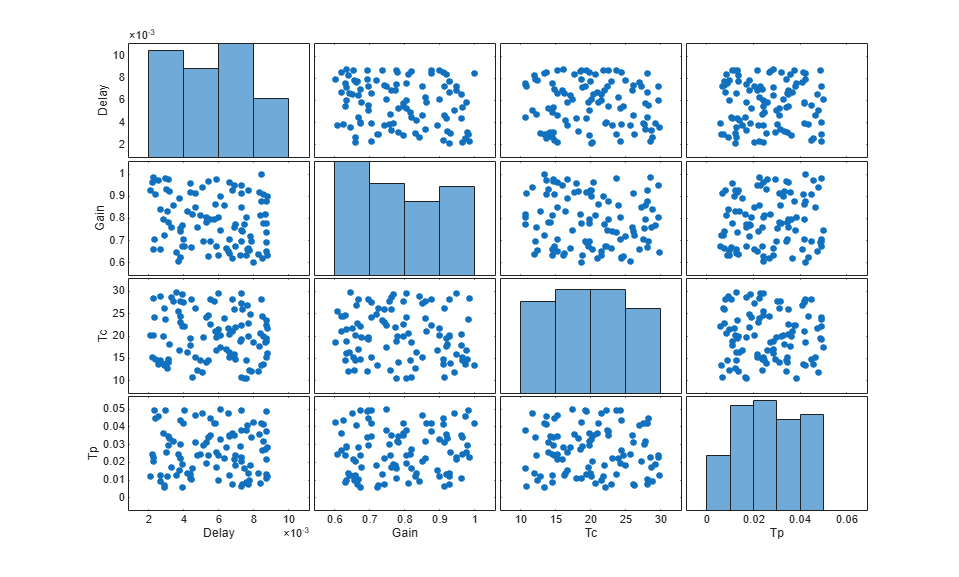The sampling above used default options, and these are reflected in the plots above. Parameter values were selected at random from distributions that were uniform over the range of each parameter. Consequently, the histogram plots along the diagonal appear approximately uniform. If Statistics and Machine Learning Toolbox™ is available, many other distributions may be used, and sampling can be done using Sobol or Halton low-discrepancy sequences.

The off-diagonal plots above show scatter plots between pairs of different variables. Since we did not specify a RankCorrelation matrix in ps, the scatter plots do not indicate correlations. However, if parameters were believed to be correlated, this can be specified using the RankCorrelation property of `ps`.

For sensitivity analysis, it is simpler to use a scalar objective, so we will specify the sum of squared errors, "SSE":

```estFcn = @(v) sdoVOR_Objective(v, Simulator, Exp, 'SSE'); y = sdo.evaluate(estFcn, ps, x); ```
```Model evaluated at 100 samples. ```

Evaluation could also be sped up using parallel computing.

Obtain the standardized regression coefficients.

```opts = sdo.AnalyzeOptions; opts.Method = 'StandardizedRegression'; sensitivities = sdo.analyze(x, y, opts); ```

Other types of analysis include correlation and, if Statistics and Machine Learning Toolbox is available, partial correlation.

We can view the analysis results.

```disp(sensitivities) ```
``` F _________ Delay 0.01303 Gain -0.90873 Tc -0.044395 Tp 0.19919 ```

For standardized regression, parameters that highly influence the model output have sensitivity magnitudes close to 1. On the other hand, less influential parameters have smaller sensitivity magnitudes. We see that this objective function is sensitive to changes in the Gain and Tp parameters, but much less sensitive to changes in the Delay and Tc parameters.

You can validate sensitivity analysis results by resampling and reevaluating the objective function for the samples. You can also use engineering intuition for a quick analysis. For example, in this model, the time constant `Tc` ranges from 10 to 30 seconds. Even the minimum value of 10 seconds is large compared to the 2-second duration for which the head motion stimulation is held at constant velocity. Therefore, `Tc` is not expected to affect the output greatly. However, even when this kind of intuition is not readily available in other models, sensitivity analysis can help highlight which parameters are influential.

Based on the results of sensitivity analysis, designate the `Delay` and `Tc` parameters as fixed when optimizing. This reduction in the number of free parameters speeds up optimization.

```Delay.Free = false; Tc.Free = false; ```

### Optimization

We can use the minimum from sensitivity analysis as the initial guess for optimization.

```[fval, idx_min] = min(y.F); Delay.Value = x.Delay(idx_min); Gain.Value = x.Gain(idx_min); Tc.Value = x.Tc(idx_min); Tp.Value = x.Tp(idx_min); % v = [Delay Gain Tc Tp]; opts = sdo.OptimizeOptions; opts.Method = 'fmincon'; ```

As was the case with model evaluations in sensitivity analysis, parallel computing could be used to speed up the optimization.

```vOpt = sdo.optimize(estFcn, v, opts); disp(vOpt) ```
``` Optimization started 26-Feb-2022 18:05:15 max First-order Iter F-count f(x) constraint Step-size optimality 0 5 13.4798 0 1 18 12.2052 0 0.129 305 2 30 11.1441 0 0.0648 790 3 41 10.0493 0 0.0843 290 4 46 9.23607 0 0.0758 286 5 51 8.76122 0 0.0183 10.1 6 56 8.75862 0 0.00184 0.476 7 57 8.75862 0 8.41e-05 0.476 Local minimum possible. Constraints satisfied. fmincon stopped because the size of the current step is less than the value of the step size tolerance and constraints are satisfied to within the value of the constraint tolerance. (1,1) = Name: 'Delay' Value: 0.0038 Minimum: 0.0020 Maximum: 0.0090 Free: 0 Scale: 0.0078 Info: [1x1 struct] (1,2) = Name: 'Gain' Value: 0.9012 Minimum: 0.6000 Maximum: 1 Free: 1 Scale: 1 Info: [1x1 struct] (1,3) = Name: 'Tc' Value: 16.6833 Minimum: 10 Maximum: 30 Free: 0 Scale: 16 Info: [1x1 struct] (1,4) = Name: 'Tp' Value: 0.0157 Minimum: 0.0050 Maximum: 0.0500 Free: 1 Scale: 0.0156 Info: [1x1 struct] 1x4 param.Continuous ```

### Visualizing Result of Optimization

Obtain the model response after estimation. Search for the model_residual signal in the logged simulation data.

```Exp = setEstimatedValues(Exp, vOpt); Simulator = createSimulator(Exp,Simulator); Simulator = sim(Simulator); SimLog = find(Simulator.LoggedData, ... get_param(model_name, 'SignalLoggingName') ); EyeSignal = find(SimLog, 'EyeMotion'); ```

Comparing the measured eye data with the optimized model response shows that the residuals are much smaller.

```estFcn = @(v) sdoVOR_Objective(v, Simulator, Exp, 'Residuals'); Model_Error = estFcn(vOpt); plot(Time, EyeData, '-g', ... EyeSignal.Values.Time, EyeSignal.Values.Data, '--c', ... Time, Model_Error.F, '-r'); xlabel('Time (sec)'); ylabel('Angular Velocity (deg/sec)'); legend('Eye Data', 'Model', 'Residual'); ```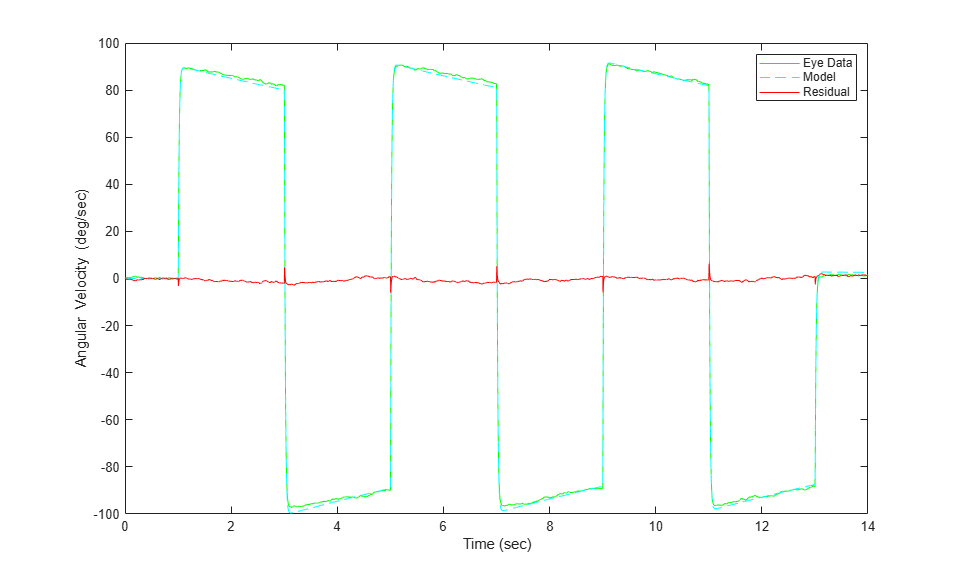Close the model.

```bdclose(model_name) ```